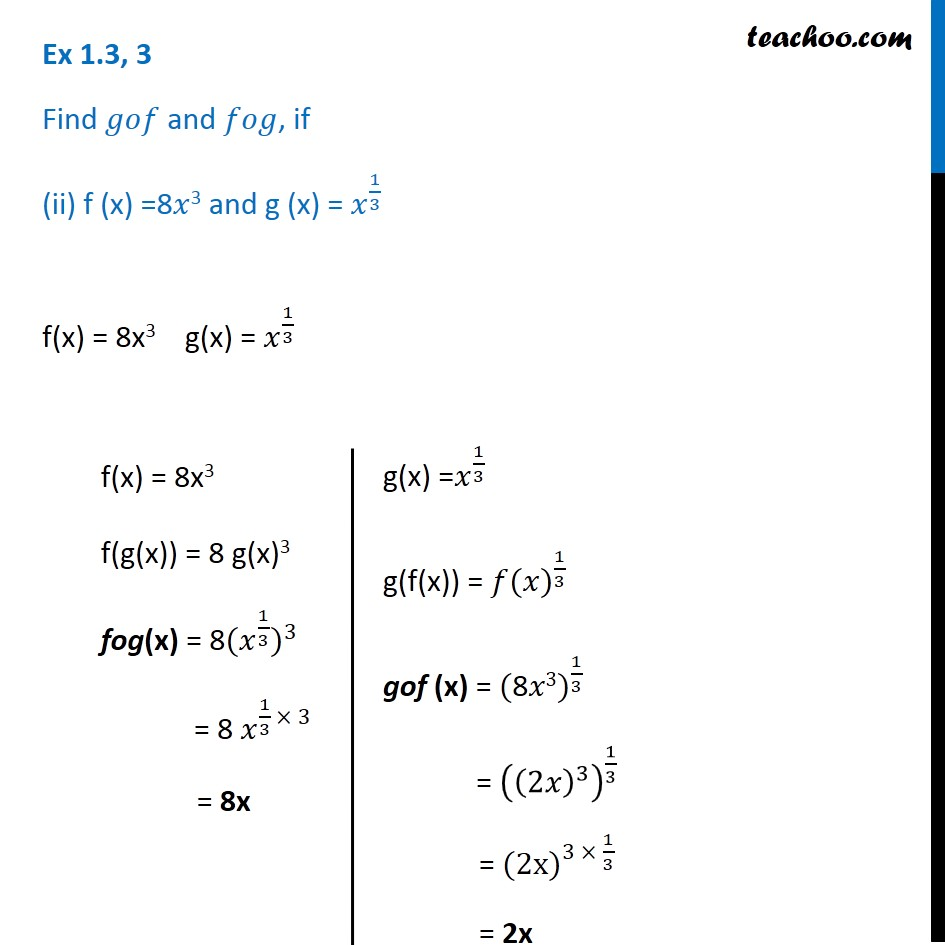Inverse of a function

Chapter 1 Class 12 Relation and Functions
Serial order wiseLearn in your speed, with individual attention - Teachoo Maths 1-on-1 Class

### Transcript

Ex 1.3, 3 Find 𝑔𝑜𝑓 and 𝑓𝑜𝑔, if (ii) f (x) =8𝑥3 and g (x) = 𝑥^(1/3) f(x) = 8x3 g(x) = 𝑥^(1/3) f(x) = 8x3 f(g(x)) = 8 g(x)3 fog(x) = 〖"8" 〖(𝑥〗^(1/3))〗^3 = 8 𝑥^(1/3 × 3) = 8x g(x) =𝑥^(1/3) g(f(x)) = 〖𝑓(𝑥)〗^(1/3) gof (x) = 〖"(8" 𝑥3)〗^(1/3) = ((2𝑥)^3 )^(1/3) = 〖"(2x" )〗^(3 × 1/3) = 2x